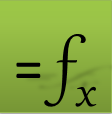## Criterion Functions Overview

A criterion function enables you to define a simple constraint formula on an array solution obtained by any of the differential equation’s solvers. A constraint formula typically computes the difference between a value from your obtained solution and your desired value. Once you have defined all necessary constraint formulas, you can use NLSOLVE to solve the system of constraints formulas to obtain optimal values for the model parameters which can include any parameters that influence the system behavior.

### How to Specify a Criterion Constraint for NLSOLVE

NLSOLVE interprets (equality and inequality) constraints formulas in relation to zero. You can specify a criterion constraint formula in the following form:

= Criterion function value – Target Value

## Quick Examples

Let the array below represent the results of an initial value problem simulation using IVSOLVE.

 A B C 1 Time Disp Velocity 2 0 1 0 3 0.1 0.95711 -0.26427 4 0.2 0.846225 -0.4425 5 0.3 0.692986 -0.54106 6 0.4 0.520265 -0.57127 .. 41 3.9 -0.00483 0.004681 42 4.0 -0.00339 0.004634

to constrain the value in B5 (which corresponds to the value of Disp at Time =0.3 to be 0.5.

=DYNVAL(B5) - 0.5

to constrain the value of the variable Disp at Time=0.35 to be 0.6.

=INTERPXY(A2:A12, DYNVAL(B2:B12), 0.35) – 0.6

In a parametric optimization, NLSOLVE will find optimal values for your design parameters that will drive your defined constraints to zero.

Question or Comment? Email us:
support @ excel-works.comExceLab: Transforming Excel into a Calculus Power House

ExceLab functions and methods are protected by USA Patents 10628634, 10114812, 9892108 and 9286286.